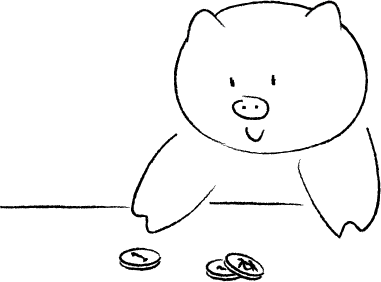# 能够连续扔出几个正面呢？HHH, HHT, HTH, HTT, THH, THT, TTH, TTT

0 1/8
1 1/2
2 1/4
3 1/8

1. 先扔一个反面，然后最多不超过三个连续正面；
2. 先扔一个正面，一个反面，然后最多不超过三个连续正面；
3. 先扔两个正面，一个反面，然后最多不超过三个连续正面；
4. 先扔三个正面，一个反面，然后最多不超过三个连续正面

$A_n(3) = A_{n-1}(3)+A_{n-2}(3)+A_{n-3}(3)+A_{n-4}(3)$

$A_n(3) = \begin{cases} \sum\limits_{j=0}^x A_{n-j-1}(x), & n > x;\\ 2^n, & n< x. \end{cases}$

$R_n \approx \log_2 n – 1$

 Schilling, Mark F. “The longest run of heads.” College Math. J 21.3 (1990): 196-207.
 Weisstein, Eric W. “Run.” From MathWorld–A Wolfram Web Resource. https://mathworld.wolfram.com/Run.html
 Révész, P. “Strong theorems on coin tossing.” Proceedings of the International Congress of Mathematicians, Helsinki. 1978.